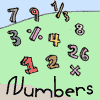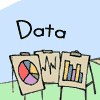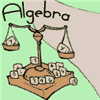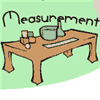# "Interactives"

Also called "Manipulatives"

Here is a collection of pages that can help you learn by doing.
There are also many Calculators and Math Games as well.

Numbers### CountingColorable Number ChartNumber Block PuzzlesNumber Blocks FreeplayChoose the Larger Block GameSkip Counting

### NumbersColorable Number ChartIn OrderOrdering GameWord Questions - General ElementaryPrime Factorization ToolScientific CalculatorMath Match GameNumber Block PuzzlesNumber Blocks FreeplaySmart BlocksWord Questions - Elementary AdditionSpeed MathReaction MathFix the EquationMath Trainer - Addition

### SubtractionWord Questions - Elementary SubtractionReaction MathFix the EquationMath Trainer - Subtraction

### MultiplicationMath Match GameTimes Table Tests From 2 to 15Speed MathReaction MathFix the EquationMath Trainer - Multiplication

### DivisionFix the Equation

### FractionsCompare FractionsInteractive FractionsLeast Common Multiple CalculatorGreatest Common Factor CalculatorOrdering Game

### PercentagesPercentage Calculator

### DecimalsOrdering Game

Data### DataThe Mean MachineData Graphs (Bar, Line, Pie)QuincunxRandom WordsStandard Deviation Calculator

Algebra### Pre-AlgebraCompare Numbers to 10Compare Numbers to 100Compare Unit FractionsCompare Decimal Numbers 0 to 1Pattern Match - ColorsPattern Match - NumbersPattern Match - ShapesShapes Freeplay

### AlgebraCalculate the Straight Line GraphSmart BlocksFunction Grapher and CalculatorVector Calculator

### Linear EquationsExplore the Straight Line GraphCalculate the Straight Line GraphExplore the Quadratic Equation GraphQuadratic Equation Solver

### TrigonometryInteractive Unit CircleFunction Grapher and CalculatorTriangle Solving Practice

Geometry### Geometry (Plane)Shapes FreeplayMath Match GameInteractive TrianglesInteractive QuadrilateralsInteractive PolygonsInteractive Cartesian Coordinates

Measurement### MeasurementInteractive ThermometerUnit Converter

### TimeAnalog and Digital Clocks AnimationHow Many Days Between Two Dates?Stopwatches